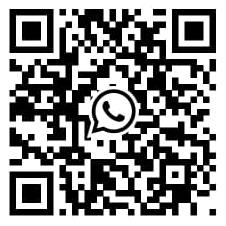# Paired Sample Test

## What is Paired Sample Test?

The article focuses on understanding Paired Sample Test, which is utilised for the statistical analysis to evaluate the data sets. In SPSS, it is possible to conduct paired T-test, where the Paired Samples T-Test mainly compares the means of two measurements, taken from the same individual, object, or related units. These "paired" measurements can be represented things like a measurement taken at two different times (e.g., pre-test and post-test score with an intervention administered at the two different time points). Hence, the paired data T-test is beneficial to analyse different data set gathered from diverse sources at different points in time.

## Interpretation

The paired sample test considers the analysis of the quantitative outcome based on the aired sample. For example, pre-test and post-test results are collected from the same individual and it is known as a paired database which is being tested through paired T-test. Cross-over trials in which the individuals are randomised to two treatments and then the same individuals are crossed over to the alternative treatment are also considered as a paired set. A matched sample is considered as a paired test where the individuals are matched on personal characteristics such as age and gender. Duplicate measurements on the same biological samples and any circumstance in which each data point in one sample is uniquely matched to a data point in the second sample. It is hereby possible to collect the aired data set on different criteria of the data and variables when the individual is the same for two cases.

Tstat = (observed mean difference − expected mean difference when 𝐻𝐻0 true) / SEMd

P-value corresponds to the AUC in the tails beyond the –|tstat| and +|tstat|, where the paired T-test is quantitative data analysis. A paired t-test is utilised, when the researchers or data analysts are interested in the difference between two variables for the same subject. Often the two variables are separated by time or based on different criteria. If a difference between two means is significant, it is considered to be paired test where the researchers try to analyse the variance between two different variables in the data set. The Paired-samples T-tests compare the scores on the two different variables, but for the same group of cases. Here, the independent-samples T-tests are helpful to compare the scores on the same variable, but for two different groups of cases.

## For interpreting the paired t-test, the steps are such as,

### Step 1: Determining a confidence interval for the population mean the difference

The confidence interval provides a range of likely values for the particular population mean difference. For example, a 95% confidence level indicates that, if the researchers take 100 random samples from the population, it could be expected approximately 95 of the samples are significant to produce intervals that contain the population mean difference.

### Step 2: Determining whether the difference is statistically significant

P-value ≤ α means the difference between the means is statistically significant and hence rejecting the null hypothesis. The alternative hypothesis is accepted as the mean-variance between the variables is significant in the data set. On the other hand, P-value > α refers to the situation that, the difference between the means is not statistically significant and hence the researchers fail to reject the null hypothesis.

### Step 3: Check your data for problems

Checking the data and evaluating to conclude is important to test the hypothesis through paired T-test.

Hence, the paired t-test is effective when the data set includes different characterises of data for the same individual. It is important to test the hypothesis to analyse the significance level of the paired database.

Finish strong by learning how our dissertation specialists and SPSS help the team support your efforts to take you to the finish line. SPSS tutor assists you with your quantitative analysis by developing your methodology and results in chapters.

You can also send us an email   or call us directly on, (+44) 20 3287 0255 and will get in touch with you shortly

I had to submit a big report within a few days. I thought it was impossible to do so. But experts from SPSS Tutor Saved the day.

Paul

had complex research about a medical condition. It was difficult to finish the tasks. But thanks to the SPSS tutor, they helped me to finish tasks on time and made it look easy.

Emily

I had to write an assignment for an IT topic. It required research on new technology. Finding information about it was difficult because it is new. But the SPSS tutor team helped me with it and today I got the highest grades in the class.

Andrew

They helped me with my statistics homework. The team is friendly, the service is nice and I liked how they kept asking me suggestions. I loved their service and would recommend it to others.

Kimberly
; ?>

Whatsapp Scan